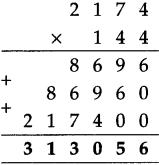# Maharashtra Board Class 5 Maths Solutions Chapter 4 Multiplication and Division Problem Set 14

Balbharti Maharashtra Board Class 5 Maths Solutions Chapter 4 Multiplication and Division Problem Set 14 Textbook Exercise Important Questions and Answers.

## Maharashtra State Board Class 5 Maths Solutions Chapter 4 Multiplication and Division Problem Set 14

Question 1.
Multiply the following:

(1) 327 × 92
Solution:
3 2 7
x
9 2
_____
6 5 4
+
2 9 4 3 0
3 0 0 8 4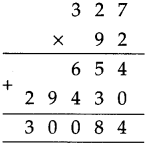(2) 807 × 126
Solution:
8 0 7
x
1 2 6
______
4 8 4 2
+
1 6 1 4 0
+
8 0 7 0 0
1 0 1 6 8 2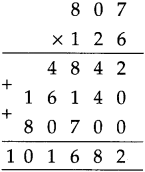(3) 567 × 890
Solution:
5 6 7
x
8 9 0
______
0 0 0
+
5 1 0 3 0
+
4 5 3 6 0 0
5 0 4 6 3 0(4) 4317 × 824
Solution:
4 3 1 7
8 2 4
1 7 2 6 8
+ 8 6 3 4 0
3 4 5 3 6 0 0
3 5 5 7 2 0 8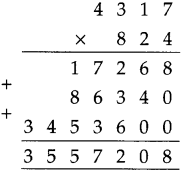(5) 6092 × 203
Solution:
6 0 9 2
x
2 0 3
______
1 8 2 7 6
+
0 0 0 0 0
+
1 2 1 8 4 0 0
1 2 3 6 6 7 6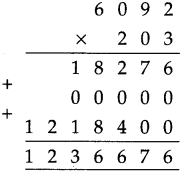(6) 1177 × 99
Solution:
1 1 7 7
x
9 9
1 0 5 9 3
+
1 0 5 9 3 0
1 1 6 5 2 3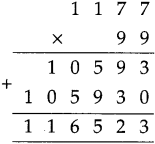(7) 456 × 187
Solution:
4 5 6
x
1 8 7
3 1 9 2
+
3 6 4 8 0
+
4 5 6 0 0
8 5 2 7 2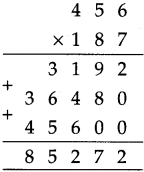(8) 6543 × 79
Solution:
6 5 4 3
x
7 9
5 8 8 8 7
+
4 5 8 0 1 0
5 1 6 8 9 7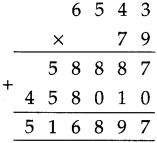(9) 2306 × 832
Solution:
2 3 0 6
x
8 3 2
______
4 6 1 2
+
6 9 1 8 0
+
1 8 4 4 8 0 0
______
1 9 1 8 5 9 2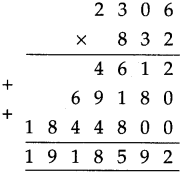(10) 6429 × 509
Solution:
6 4 2 9
x
5 0 9
______
5 7 8 6 1
+
0 0 0 0 0
+
3 2 1 4 5 0 0
3 2 7 2 3 6 1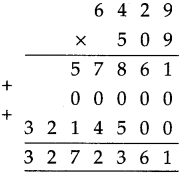(11) 4,321 × 678
Solution:
4 3 2 1
x
6 7 8
_____
3 4 5 6 8
3 0 2 4 7 0
2 5 9 2 6 0 0
_______
2 9 2 9 6 3 8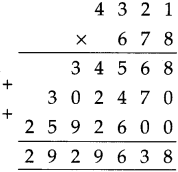(12) 20,304 × 87
Solution:
2 0 3 0 4
x
8 7
1 4 2 1 2 8
+
1 6 2 4 3 2 0
_________
1 7 6 6 4 4 8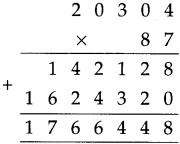Question 2.
As part of the ‘Avoid Plastic’ campaign, each of 745 students made 25 paper bags. What was the total number of paper bags made ?
Solution:
7 4 5 Number of students
x
2 5 bags made by each
___________
3 7 2 5
+
1 4 9 0 0
__________
1 8 6 2 5
__________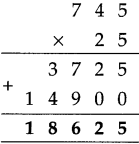Question 3.
In a plantation, saplings of 215 medicinal trees have been planted in each of the 132 rows of trees. How many saplings are there in the plantation altogether ?
Solution:
2 1 5 Saplings in each now
x
1 3 2 Number of rows
__________
4 3 0
+
6 4 5 0
+
2 1 5 0 0
__________
2 8 3 8 0
__________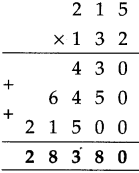Altogether there is 28,380 saplings.

Question 4.
One computer costs 27,540 rupees. How much will 18 such computers cost ?

Solution:
2 7 5 4 0 Cost of 1 computer
x
1 8 No. of computers
__________
2 2 0 3 2 0
+
2 7 5 4 0 0
___________
4 9 5 7 2 0
__________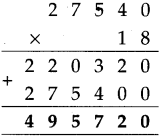4,95,720 rupees cost of 18 computers.

Question 5.
Under the ‘Inspire Awards’ scheme, 5000 rupees per student were granted for the purchase of science project materials. If 154 students in a certain taluka were covered under the scheme, find the total amount granted to that taluka.

Solution:
₹ 5 0 0 0 Granted per student
x 1 5 4 Number of students
__________
2 0 0 0 0
+
2 5 0 0 0 0
+
5 0 0 0 0 0
______________
₹ 7 7 0 0 0 0
______________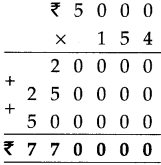7,70,0000 granted totally

Question 6.
If a certain two-wheeler costs 53,670 rupees, how much will 35 such two-wheelers cost ?
Solution:
5 3 7 6 0 Cost of 1 two-wheeler
x 3 5 No. of two-wheelers
___________
2 6 8 8 0 0
+
1 6 1 2 8 0 0
_____________
1 8 8 1 6 0 0
______________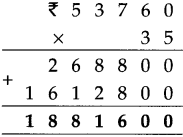18,81,600 is the total cost of 35- two-wheelers

Question 7.
One hour has 3,600 seconds. How many seconds do 365 hours have ?
Solution:
3 6 0 0 Seconds of 1 hour
x
3 6 5 No. of hours
_________
1 8 0 0 0
+
2 1 6 0 0 0
+
1 0 8 0 0 0 0
______________
1 3 1 4 0 0 0
______________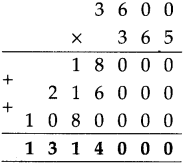13,14,000 seconds for 365 hours.

Question 8.
Frame a multiplication word problem with the numbers 5473 and 627 and solve it.

Solution:
Cost of one mobile is 5,473. What is the cost of such 627 mobiles?
5 4 7 3 Cost of 1 mobile
x 6 2 7 Number of mobiles
__________
3 8 3 1 1
+
1 0 9 4 6 0
+
3 2 8 3 8 0 0
_____________
3 4 3 1 5 7 1
_____________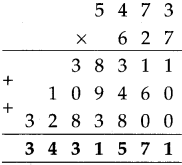34,31,571 cost for 627 mobiles.

Question 9.
Find the product of the biggest three-digit number and the biggest four-digit number.

Solution:
9 9 9 9 Biggest four digit no.
x 9 9 9 Biggest three-digit no.
_________
8 9 9 9 1
+
8 9 9 9 1 0
+
8 9 9 9 1 0 0
_____________
9 9 8 9 0 0 1
_____________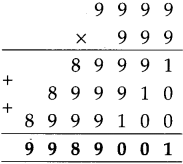99,89,001

Question 10.
One traveller incurs a cost of 7,650 rupees for a certain journey. What will be the cost for 26 such travellers?
Solution:
7 6 5 0 Cost of one traveller
x 2 6 No. of travellers
______
4 5 9 0 0
+
1 5 3 0 0 0
_____________
1 9 8 9 0 0
_____________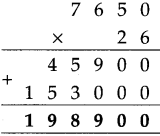1,98,900 cost of 26 travellers.

Pairing off objects from two groups in different ways

(1) Ajay wants to travel light. So he took with him three shirts – one red, one green and one blue and two pairs of trousers – one white and one black. How many different ways does he have of pairing off a shirt with trousers?

Writing ‘S’ for shirt and ‘T’ for trousers, the possible different pairs are :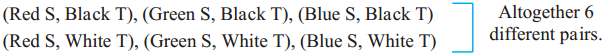(2) Suresh has three balls of different colours marked A, B and C and three bats marked P, Q and R. He wishes to take only one bat and one ball to the playground. In how many ways can he pair off a ball and a bat to take with him?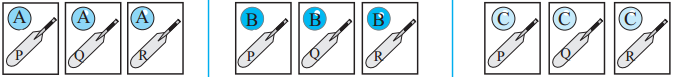How many different pairs have been shown here?

(3) The three friends, Sanju, John and Ali went to the fair. A shop there, had five different types of hats. Each of the boys had photos taken of himself, wearing every type of hat, in turn. Find how many photographs were taken in all.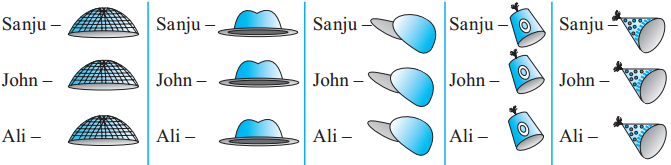How many different pairs were formed ? That is, how many photos were taken ?

Take two collections, each containing the given number of objects. Make as many different pairs as possible, taking one object from each collection every time. Thus, complete the table below.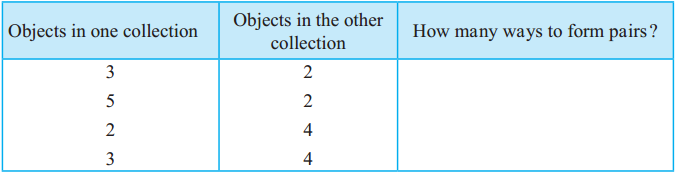What does this table tell us ?
The number of different pairs formed by pairing off objects from two groups is equal to the product of the number of objects in the two groups.

Division
Teacher : You have learnt some things about division. For example, we know that division means making equal parts of a given number, or, subtracting a number repeatedly from a given number. What else do you know ?
Shubha : We know that we get two divisions from one multiplication. From 9 × 4 = 36, we get the divisions 36 ÷ 4 = 9 and 36 ÷ 9 = 4.
Teacher : Very good! Right now, there’s nothing new to learn about division. Only the number of digits in the dividend and the divisor will grow. Tell me what is 354 ÷ 6 ?
Sarang : 354 = 300 + 54. 300 divided by six is 50. And 54 ÷ 6 = 9. Hence the quotient is 50 + 9 = 59.
Teacher : Right! Now let’s learn, step by step, how to divide a four-digit number by a one-digit number. So now, divide 4925 by 7 and tell me the quotient and the remainder.
Shubha : We cannot divide 4 thousands by 7 into whole thousands. Now, 4 Th = 40 H. So let us instead take the 40 hundreds together with 9 hundreds and divide 49 hundreds. 49 ÷ 7 = 7. So, everyone gets 7 hundreds. Now, we cannot divide 2T equally among 7 people. So we must write 0 in the tens place in the quotient. Then on dividing 25 by seven, we get quotient 3 and the remainder is 4. Thus, the answer is quotient 703, remainder 4.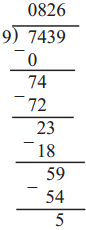Teacher : Very good ! Now divide 7439 by 9.
Sarang : It’s difficult to do this mentally. I’ll write it down on paper. The quotient is 826 and the remainder, 5.
Teacher : We use the same method to divide a four-digit number by a two-digit number. If necessary, we can prepare the table of the divisor before we start.

Study the solved examples shown below.
Example (1)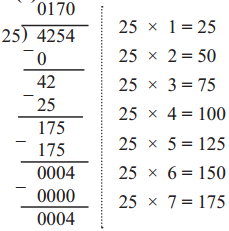Quotient 170, Remainder 4

Example (2)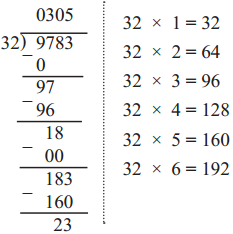Quotient 305, Remainder 23

Example (3) Divide. 9842 ÷ 45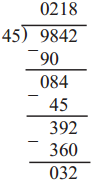We can prepare the 45 times table to do this division.
But when the divisor is a big number, we can solve the example by first guessing what the quotient will be. Let us see how to do that.
We have 0 in the thousands place in the quotient.
Now, to guess the quotient when dividing 98 by 45, look at the first digits in both – the dividend and the divisor. These are 9 and 4, respectively.
Dividing 9 by 4, we will get 2 in the quotient. Let us see if 2 times 45 can be subtracted from 98. 45 × 2 = 90. 90 < 98. So, we write 2 in the hundreds place in the quotient.
Next, dividing 84 by 45 we can easily see that as 90 > 84, we have to write 1 in the tens place in the quotient.
Now, we have to divide 392 by 45. As 3 < 4, let us look at 39, the number formed by the first 2 digits, to guess the next digit in the quotient.
4 × 9 = 36 and 36 < 39. Let us check if the next digit can be 9. 45 × 9 = 405 and 405 > 392. Therefore, 9 cannot be the next digit in the quotient.
Let us check for 8. 45 × 8 = 360. 360 < 392. So, we write 8 in the units place of the quotient.
We subtract 8 × 45 from 392 and complete the division.
The quotient is 218 and the remainder, 32.

Example (4)
If 35 kilograms of wheat cost 910 rupees, what is the rate of wheat per kg?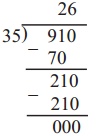Weight of wheat in kg × rate of wheat per kg = cost of wheat Hence, 35 × rate per kg = 910
Therefore, when we divide 910 by 35, we will get the per kg rate of wheat.
The rate per kilogram of wheat is 26 rupees.

Multiply the following:

(1) 2132 x 231
Solution:
2 1 3 2
x
2 3 1
2 1 3 2
+
6 3 9 6 0
+
4 2 6 4 0 0
____________
4 9 2 4 9 2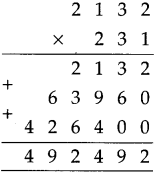(2) 1863 x 432
Solution:
1 8 6 3
x
4 3 2
3 7 2 6
+
5 5 8 9 0
+
7 4 5 2 0 0
___________
8 0 4 8 1 6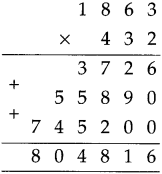Solve the following word problems:

(1) A factory manufactures 34,796 pairs of socks in one hour. How many pairs will the factory manufacture in one day?
Solution:
3 4 7 9 6 Pairs of socks
x 2 4 No. of hours
______
1 3 9 1 8 4
+
6 9 5 9. 2 0
_____________
8 3 5 1 0 4
_____________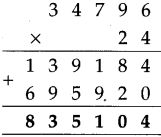8,35,104 pairs of socks manufactured in one day

(2) There are 375 toffees in a box. How many toffees will be there in 632 such boxes?
Solution:
3 7 5 No. of toffees
x 6 3 2 No. of boxes
_______
7 5 0
+
1 1 2 5 0
+
2 2 5 0 0 0
___________
2 3 7 0 0 0
_____________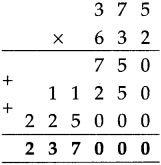There will be 2,37,000 toffees.

(3) There are 144 articles in a gross. How many articles are there in 2174 gross?
Solution:
2 1 7 4 No. of gross
x 1 4 4 Articles in I gross
______
8 6 9 6
+
8 6 9 6 0
+
2 1 7 4 0 0
_____________
3 1 3 0 5 6
_____________